## Producer Field Guide

HGD_Product
Producer Field Guide
HGD_Portfolio_Suite
Producer

Radiometric enhancement deals with the individual values of the pixels in the image. It differs from spatial enhancement (discussed in Spatial Enhancement), which takes into account the values of neighboring pixels.

Depending on the points and the bands in which they appear, radiometric enhancements that are applied to one band may not be appropriate for other bands. Therefore, the radiometric enhancement of a multiband image can usually be considered as a series of independent, single-band enhancements (Faust, 1989).

Radiometric enhancement usually does not bring out the contrast of every pixel in an image. Contrast can be lost between some pixels, while gained on others.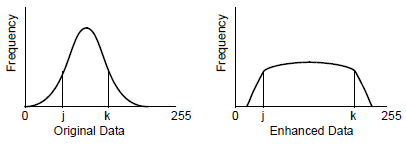In the figure above, the range between j and k in the histogram of the original data is about one third of the total range of the data. On the graph, j and k are reference points. When the same data are radiometrically enhanced, the range between j and k can be widened. Therefore, the pixels between j and k gain contrast—it is easier to distinguish different brightness values in these pixels.

However, the pixels outside the range between j and k are more grouped together than in the original histogram to compensate for the stretch between j and k. Contrast among these pixels is lost.

Contrast Stretching

When radiometric enhancements are performed on the display device, the transformation of data file values into brightness values is illustrated by the graph of a lookup table.

For example, the figure below shows the graph of a lookup table that increases the contrast of data file values in the middle range of the input data (the range within the brackets). Note that the input range within the bracket is narrow, but the output brightness values for the same pixels are stretched over a wider range. This process is called contrast stretching.

Graph of a Lookup Table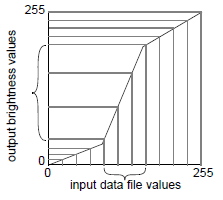Notice that the graph line with the steepest (highest) slope brings out the most contrast by stretching output values farther apart.

Linear and Nonlinear

The terms linear and nonlinear, when describing types of spectral enhancement, refer to the function that is applied to the data to perform the enhancement. A piecewise linear stretch uses a polyline function to increase contrast to varying degrees over different ranges of the data, as in the figure below.

Enhancement with Lookup Tables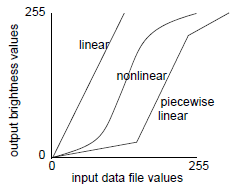Linear Contrast Stretch

A linear contrast stretch is a simple way to improve the visible contrast of an image. It is often necessary to contrast-stretch raw image data, so that they can be seen on the display.

In most raw data, the data file values fall within a narrow range—usually a range much narrower than the display device is capable of displaying. That range can be expanded to utilize the total range of the display device (usually 0 to 255).

A Percentage LUT with clip of 2.5% from left end and 1.0% from right end of the histogram linear contrast stretch is automatically applied to images displayed in the Viewer.

Nonlinear Contrast Stretch

A nonlinear spectral enhancement can be used to gradually increase or decrease contrast over a range, instead of applying the same amount of contrast (slope) across the entire image. Usually, nonlinear enhancements bring out the contrast in one range while decreasing the contrast in other ranges. The graph of the function in the figure in the next section below shows one example.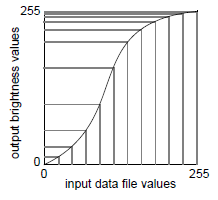Piecewise Linear Contrast Stretch

A piecewise linear contrast stretch allows for the enhancement of a specific portion of data by dividing the lookup table into three sections: low, middle, and high. You can create a number of straight line segments that can simulate a curve. You can enhance the contrast or brightness of any section in a single color gun at a time. This technique is very useful for enhancing image areas in shadow or other areas of low contrast.

In ERDAS IMAGINE, the Piecewise Linear Contrast function is set up so that there are always pixels in each data file value from 0 to 255. You can manipulate the percentage of pixels in a particular range, but you cannot eliminate a range of data file values.

A piecewise linear contrast stretch normally follows two rules:

1. Data values are continuous; there can be no break in the values between High, Middle, and Low. Range specifications adjust in relation to any changes to maintain the data value range.

2. Data values specified can go only in an upward, increasing direction, as shown in the figure below.

Piecewise Linear Contrast Stretch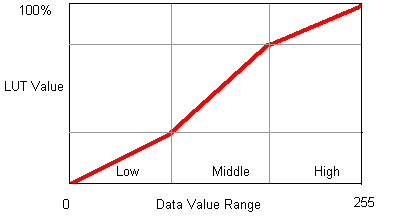The contrast value for each range represents the percent of the available output range that particular range occupies. The brightness value for each range represents the middle of the total range of brightness values occupied by that range. Since rules 1 and 2 above are enforced, as the contrast and brightness values are changed, they may affect the contrast and brightness of other ranges. For example, if the contrast of the low range increases, it forces the contrast of the middle to decrease.

Contrast Stretch on the Display

Usually, a contrast stretch is performed on the display device only, so that the data file values are not changed. Lookup tables are created that convert the range of data file values to the maximum range of the display device. You can then edit and save the contrast stretch values and lookup tables as part of the raster data image file. These values are loaded into the Viewer as the default display values the next time the image is displayed.

In ERDAS IMAGINE, you can permanently change the data file values to the lookup table values. Use the LUT Stretch function to create an .img output file with the same data values as the displayed contrast stretched image.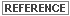See Raster Data for more information on the data contained in image files.

The statistics in the image file contain the mean, standard deviation, and other statistics on each band of data. The mean and standard deviation are used to determine the range of data file values to be translated into brightness values or new data file values. You can specify the number of standard deviations from the mean that are to be used in the contrast stretch. Usually the data file values that are two standard deviations above and below the mean are used. If the data have a normal distribution, then this range represents approximately 95 percent of the data.

The mean and standard deviation are used instead of the minimum and maximum data file values because the minimum and maximum data file values are usually not representative of most of the data. A notable exception occurs when the feature being sought is in shadow. The shadow pixels are usually at the low extreme of the data file values, outside the range of two standard deviations from the mean.Using these statistics in contrast stretching is discussed and illustrated in Image Display. Statistical terms are discussed in Math Topics.

Varying the Contrast Stretch

There are variations of the contrast stretch that can be used to change the contrast of values over a specific range, or by a specific amount. By manipulating the lookup tables as in the figure below, the maximum contrast in the features of an image can be brought out.

The figure below shows how the contrast stretch manipulates the histogram of the data, increasing contrast in some areas and decreasing it in others. This is also a good example of a piecewise linear contrast stretch, which is created by adding breakpoints to the histogram. In the Figure:

1. Linear stretch. Values are clipped at 255.

2. A breakpoint is added to the linear function, redistributing the contrast.

3. Another breakpoint is added. Contrast at the peak of the histogram continues to increase.

4. Breakpoint at the top of the function is moved so that values are not clipped.

Contrast Stretch Using Lookup Tables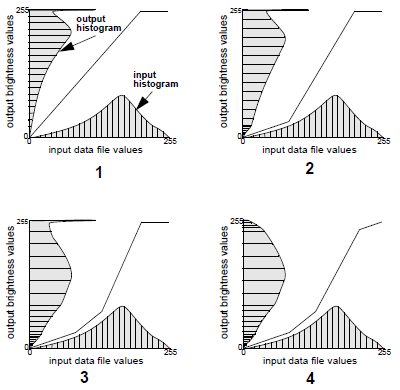Histogram Equalization

Histogram equalization is a nonlinear stretch that redistributes pixel values so that there is approximately the same number of pixels with each value within a range. The result approximates a flat histogram. Therefore, contrast is increased at the peaks of the histogram and lessened at the tails.

Histogram equalization can also separate pixels into distinct groups if there are few output values over a wide range. This can have the visual effect of a crude classification.

Histogram Equalization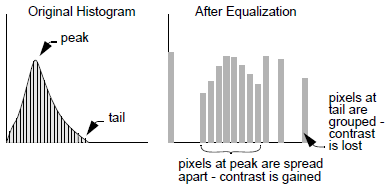To perform a histogram equalization, the pixel values of an image (either data file values or brightness values) are reassigned to a certain number of bins, which are simply numbered sets of pixels. The pixels are then given new values, based upon the bins to which they are assigned.

The following parameters are entered:

• N - number of bins to which pixel values can be assigned. If there are many bins or many pixels with the same value or values, some bins may be empty.
• M - maximum of the range of the output values. The range of the output values is from 0 to M.

The total number of pixels is divided by the number of bins, equaling the number of pixels per bin, as shown in the following equation: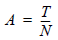Where:

N = number of bins

T = total number of pixels in the image

A = equalized number of pixels per bin

The pixels of each input value are assigned to bins, so that the number of pixels in each bin is as close to A as possible. Consider the figure below:

Histogram Equalization Example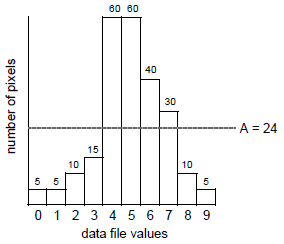There are 240 pixels represented by this histogram. To equalize this histogram to 10 bins, there would be:

240 pixels / 10 bins = 24 pixels per bin = A

To assign pixels to bins, the following equation is used: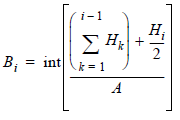Where:

A = equalized number of pixels per bin (see above)

Hi = number of values with the value i (histogram)

int = integer function (truncating real numbers to integer)

Bi = bin number for pixels with value i

Source: Modified from Gonzalez and Wintz, 1977

The 10 bins are rescaled to the range 0 to M. In this example, M = 9, because the input values ranged from 0 to 9, so that the equalized histogram can be compared to the original. The output histogram of this equalized image looks like the figure below:

Equalized Histogram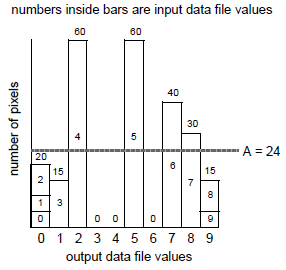Effect on Contrast

By comparing the original histogram of the example data with the one above, you can see that the enhanced image gains contrast in the peaks of the original histogram. For example, the input range of 3 to 7 is stretched to the range 1 to 8. However, data values at the tails of the original histogram are grouped together. Input values 0 through 2 all have the output value of 0. So, contrast among the tail pixels, which usually make up the darkest and brightest regions of the input image, is lost.

The resulting histogram is not exactly flat, since the pixels can rarely be grouped together into bins with an equal number of pixels. Sets of pixels with the same value are never split up to form equal bins.

Level Slice

A level slice is similar to a histogram equalization in that it divides the data into equal amounts. A level slice on a true color display creates a stair-stepped lookup table. The effect on the data is that input file values are grouped together at regular intervals into a discrete number of levels, each with one output brightness value.

To perform a true color level slice, you must specify a range for output brightness values and a number of output levels. The lookup table is then stair-stepped so that there is an equal number of input pixels in each output level.

Histogram Matching

Histogram matching is the process of determining a lookup table that converts the histogram of one image to resemble the histogram of another. Histogram matching is useful for matching data of the same or adjacent scenes that were scanned on separate days, or are slightly different because of sun angle or atmospheric effects. This is especially useful for mosaicking or change detection.

To achieve good results with histogram matching, the two input images should have similar characteristics:

• The general shape of the histogram curves should be similar.
• Relative dark and light features in the image should be the same.
• For some applications, the spatial resolution of the data should be the same.
• The relative distributions of land covers should be about the same, even when matching scenes that are not of the same area. If one image has clouds and the other does not, then the clouds should be removed before matching the histograms. This can be done using AOI function.

In ERDAS IMAGINE, histogram matching is performed band-to-band. For example, band 2 of one image is matched to band 2 of the other image.

To match the histograms, a lookup table is mathematically derived, which serves as a function for converting one histogram to the other, as illustrated in the figure below.

1 - Source histogram

2 - Mapped through the lookup table

3 - Approximates model histogram

Histogram Matching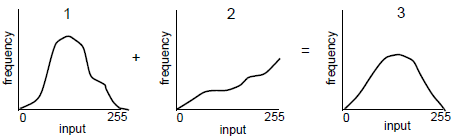Brightness Inversion

The brightness inversion functions produce images that have the opposite contrast of the original image. Dark detail becomes light, and light detail becomes dark. This can also be used to invert a negative image that has been scanned to produce a positive image.

Brightness inversion has two options: inverse and reverse. Both options convert the input data range (commonly 0 - 255) to 0 - 1.0. A min-max remapping is used to simultaneously stretch the image and handle any input bit format. The output image is in floating point format, so a min-max stretch is used to convert the output image into 8-bit format.

Inverse is useful for emphasizing detail that would otherwise be lost in the darkness of the low DN pixels. This function applies the following algorithm: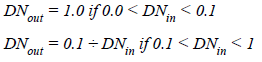Reverse is a linear function that simply reverses the DN values: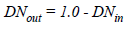Source: Pratt, 1991